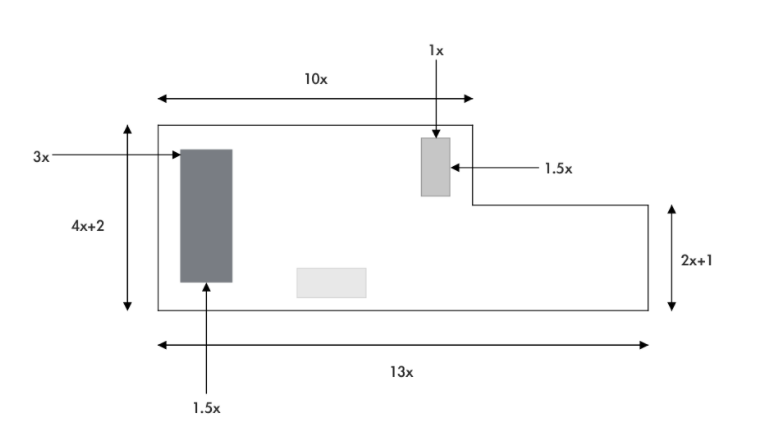¿Todavía tienes preguntas de matemáticas?

Pregunte a nuestros tutores expertos
Algebra
Question

Mr. Tortola wants to put a new coat of stain on his back patio. The following diagram shows the design of the patio, with all dimensions represented as polynomials. The main patio is a rectangle, with a smaller rectangle on the end, a hot tub (blue), and $$2$$ congruent flower boxes (grey).a) Create a simplified polynomial to represent the area of the patio that needs to be stained. Assume that the areas covered by the hot tub and the flower boxes will not be stained.

b) If the actual value of $$x=1\mathrm{m}$$, what is the area of the patio to be stained?

c) If a can of stain covers $$35\mathrm{m}^2$$ and costs $$\$$, how much will it cost Mr. Tortola to stain the patio with $$1$$ coat of stain?

a) area to be stained is $$38.5x^2+23x$$
b) $$\mathrm{A}=61.5\mathrm{m}^2$$
c) $$\87.86$$